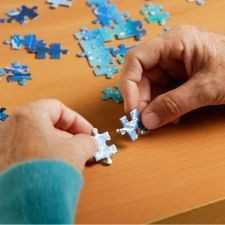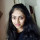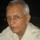Brainy Puzzles
Puzzle Solvers: Interesting brainy puzzles shared by fellow puzzlers
358 Members
Join this group to post and comment.# Fresh Puzzle for the day - Egg Puzzle

A building of 100 floors is present. You have only 2 artificial eggs made up of plastics with you. There is a one floor on the building from which this egg will break if released.It is understood that if egg is released from the floor higher than that floor then also egg will break on striking the ground.
You have to tell the minimum no. of ways in which you can find out that floor from which the egg starts breaking on hitting the ground.
Remember that you have only 2 eggs for doing this task.Frooty
A building of 100 floors is present. You have only 2 artificial eggs made up of plastics with you. There is a one floor on the building from which this egg will break if released.It is understood that if egg is released from the floor higher than that floor then also egg will break on striking the ground.
You have to tell the minimum no. of ways in which you can find out that floor from which the egg starts breaking on hitting the ground.
Remember that you have only 2 eggs for doing this task.
Since there is a lowest floor out of the hundred from where the the egg starts breaking. start with floor 1 and go on dropping the same egg from all odd numbered floors. The egg can be reused till it breaks. Let us say that you find a break at (2n + 3)rd floor and not at (2n + 1) (n varying from 0 to 49). Drop the second egg from (2n + 2)th floor. If this also breaks (2n + 2)th floor is the answer. Otherwise (2n + 3) is the answer.

Bonus: All the climbing up and down will shed unwanted pounds.Rupam Das • Sep 24, 2011
Bonus: All the climbing up and down will shed unwanted pounds.
Can we also not have real Eggs and I utilize it better than finding how it might not break. I rather have them in my body to get the energy to ride those 100 floor! if I ever can! Jokes apart, must admire Mr. Bioramani for the answer.
@Frooty can we have something simple which we can answer ?@Rupam : sure. I will post some easy going puzzle in short time 😀anyway.. this puzzle was asked in some technical interview of some IT company. Anyway I will post the answer today evening.Here is the mathematical explanation for the answer.

We have 100 floors.
Also sum of the first 'n' natural no. = n(n+1)/2
We know that sum of the first 'n' natural no. cannot be less than the no. of floors given. But the sum could be greater or equal to the no. of floors. So, we have
n(n+1)/2>_ 100 { >_ represents greater or equal to}
Aslo,n(n+1) >_200 > 182
{Inequality remains same as 200 > 182.We took 182 because it is the nearest multiple of 2 natural numbers}
Thus, n^2+n-182 >0
So, (n+14)(n-13)>0
or, n> -14, 13
We know that n should not be negative as no. of trials can't be negative.

As, n>13
Hence, n=14silverscorpion • Sep 25, 2011
Frooty
We know that sum of the first 'n' natural no. cannot be less than the no. of floors given. But the sum could be greater or equal to the no. of floors.
I don't get this line. Can you explain what this line means?Frooty
Here is the mathematical explanation for the answer.

We have 100 floors.
Also sum of the first 'n' natural no. = n(n+1)/2
We know that sum of the first 'n' natural no. cannot be less than the no. of floors given. But the sum could be greater or equal to the no. of floors. So, we have
n(n+1)/2>_ 100 { >_ represents greater or equal to}
Aslo,n(n+1) >_200 > 182
{Inequality remains same as 200 > 182.We took 182 because it is the nearest multiple of 2 natural numbers}
Thus, n^2+n-182 >0
So, (n+14)(n-13)>0
or, n> -14, 13
We know that n should not be negative as no. of trials can't be negative.

As, n>13
Hence, n=14
Something does not gel in this. Incidentally the mathematics of the above is not rigorous.

From the statement of the puzzle, the floor in question can be anything from 1 to 100 without any additonal constraint.

Let us say that the 100th floor is the one from which the egg will break if dropped. Can you please detail how this can be found with 14 trials?
Likewise if the 1st floor is itself the culprit, one trial alone can catch it.

Share this content on your social channels -